Next: Coordinate Transformations Up: Vectors and Vector Fields Previous: Vector Algebra

# Cartesian Components of a Vector

Consider a Cartesian coordinate system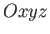, consisting of an origin,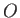, and three mutually perpendicular coordinate axes,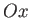,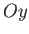, and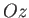. (See Figure A.4.) Such a system is said to be right-handed if, when looking along thedirection, a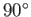clockwise rotation aboutis required to takeinto. Otherwise, it is said to be left-handed. It is conventional to always use a right-handed coordinate system.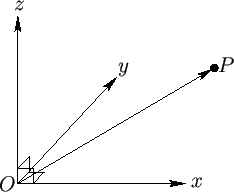It is convenient to define unit vectors,,, and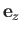, parallel to,, and, respectively. Incidentally, a unit vector is a vector whose magnitude is unity. The position vector,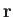, of some general point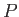whose Cartesian coordinates are (,,) is then given by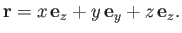(A.11)

In other words, we can get fromtoby moving a distanceparallel to, then a distanceparallel to, and then a distanceparallel to. Similarly, if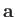is an arbitrary vector then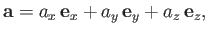(A.12)

where,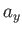, and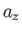are termed the Cartesian components of. It is conventional to write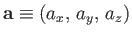. It follows that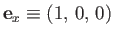,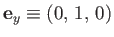, and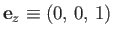. Of course,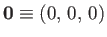.

According to the three-dimensional generalization of the Pythagorean theorem, the distance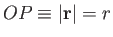is given by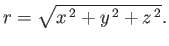(A.13)

By analogy, the magnitude of a general vectortakes the form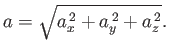(A.14)

Ifand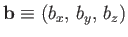then it is easily demonstrated that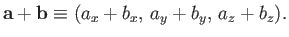(A.15)

Furthermore, if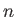is a scalar then it is apparent that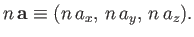(A.16)Next: Coordinate Transformations Up: Vectors and Vector Fields Previous: Vector Algebra
Richard Fitzpatrick 2016-03-31